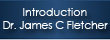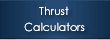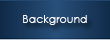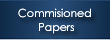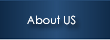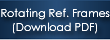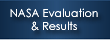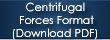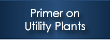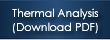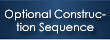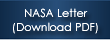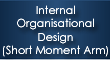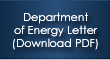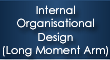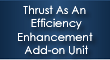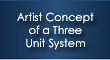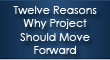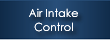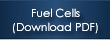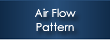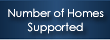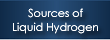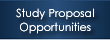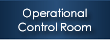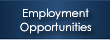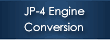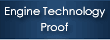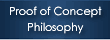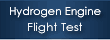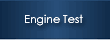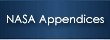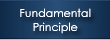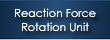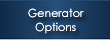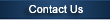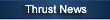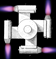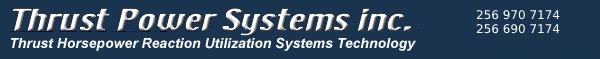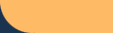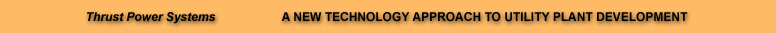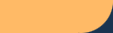Centrifugal Forces and Related Data F=mv^2/r Units r= feet, m =mass of engine v=Lower case Omega angular velocity in the units of Radians Radians are unit - less ; Velocity^2/r (Mass) = pound force To convert pound force to newtons multiply by 4.448 Click Here for discussion of Rotating Reference Frames Click Here Go Directly to Centrifugal Force Calculator